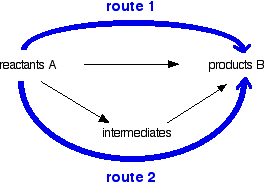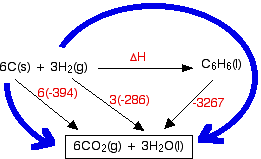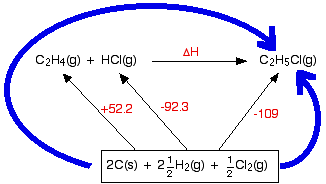HESS'S LAW AND ENTHALPY CHANGE CALCULATIONS

```
```

This page explains Hess's Law, and uses it to do some simple enthalpy change calculations involving enthalpy changes of reaction, formation and combustion.

```
```

Hess's Law

Stating Hess's Law

Hess's Law is the most important law in this part of chemistry. Most calculations follow from it. It says . . .

 The enthalpy change accompanying a chemical change is independent of the route by which the chemical change occurs.
```
```

Explaining Hess's Law

Hess's Law is saying that if you convert reactants A into products B, the overall enthalpy change will be exactly the same whether you do it in one step or two steps or however many steps.

If you look at the change on an enthalpy diagram, that is actually fairly obvious.This shows the enthalpy changes for an exothermic reaction using two different ways of getting from reactants A to products B. In one case, you do a direct conversion; in the other, you use a two-step process involving some intermediates.

In either case, the overall enthalpy change must be the same, because it is governed by the relative positions of the reactants and products on the enthalpy diagram.

If you go via the intermediates, you do have to put in some extra heat energy to start with, but you get it back again in the second stage of the reaction sequence.

However many stages the reaction is done in, ultimately the overall enthalpy change will be the same, because the positions of the reactants and products on an enthalpy diagram will always be the same.

Note:  It is possibly confusing that I am switching between the terms enthalpy and energy. Enthalpy change is simply a particular measure of energy change. You will remember that the enthalpy change is the heat evolved or absorbed during a reaction happening at constant pressure.

I have labelled the vertical scale on this particular diagram as enthalpy rather than energy, because we are specifically thinking about enthalpy changes. I could have just kept to the more general term "energy", but I prefer to be accurate.

You can do calculations by setting them out as enthalpy diagrams as above, but there is a much simpler way of doing it which needs virtually no thought.

You could set out the above diagram as:Hess's Law says that the overall enthalpy change in these two routes will be the same. That means that if you already know two of the values of enthalpy change for the three separate reactions shown on this diagram (the three black arrows), you can easily calculate the third - as you will see below.

The big advantage of doing it this way is that you don't have to worry about the relative positions of everything on an enthalpy diagram. It is completely irrelevant whether a particular enthalpy change is positive or negative.

Warnings!

Although most calculations you will come across will fit into a triangular diagram like the above, you may also come across other slightly more complex cases needing more steps. That doesn't make it any harder!

You need to take care in choosing your two routes. The pattern will not always look like the one above. You will see that in the examples below.

```
```

Enthalpy change calculations using Hess's Law cycles

I can only give a brief introduction here, because this is covered in careful, step-by-step detail in my chemistry calculations book.

```
```

Working out an enthalpy change of formation from enthalpy changes of combustion

If you have read an earlier page in this section, you may remember that I mentioned that the standard enthalpy change of formation of benzene was impossible to measure directly. That is because carbon and hydrogen won't react to make benzene.

Important:  If you don't know (without thinking about it too much) exactly what is meant by standard enthalpy change of formation or combustion, you must get this sorted out now. Re-read the page about enthalpy change definitions before you go any further - and learn them!

Standard enthalpy changes of combustion, ΔH°c are relatively easy to measure. For benzene, carbon and hydrogen, these are:

ΔH°c (kJ mol-1)
C6H6(l)-3267
C(s)-394
H2(g)-286

First you have to design your cycle.

• Write down the enthalpy change you want to find as a simple horizontal equation, and write ΔH over the top of the arrow. (In diagrams of this sort, we often miss off the standard symbol just to avoid clutter.)

• Then fit the other information you have onto the same diagram to make a Hess's Law cycle, writing the known enthalpy changes over the arrows for each of the other changes.

• Finally, find two routes around the diagram, always going with the flow of the various arrows. You must never have one of your route arrows going in the opposite direction to one of the equation arrows underneath it.

In this case, what we are trying to find is the standard enthalpy change of formation of benzene, so that equation goes horizontally.```
```

You will notice that I haven't bothered to include the oxygen that the various things are burning in. The amount of oxygen isn't critical because you just use an excess anyway, and including it really confuses the diagram.

Why have I drawn a box around the carbon dioxide and water at the bottom of the cycle? I tend to do this if I can't get all the arrows to point to exactly the right things. In this case, there is no obvious way of getting the arrow from the benzene to point at both the carbon dioxide and the water. Drawing the box isn't essential - I just find that it helps me to see what is going on more easily.

Notice that you may have to multiply the figures you are using. For example, standard enthalpy changes of combustion start with 1 mole of the substance you are burning. In this case, the equations need you to burn 6 moles of carbon, and 3 moles of hydrogen molecules. Forgetting to do this is probably the most common mistake you are likely to make.

How were the two routes chosen? Remember that you have to go with the flow of the arrows. Choose your starting point as the corner that only has arrows leaving from it. Choose your end point as the corner which only has arrows arriving.

Now do the calculation:

Hess's Law says that the enthalpy changes on the two routes are the same. That means that:

ΔH - 3267 = 6(-394) + 3(-286)

Rearranging and solving:

ΔH = 3267 + 6(-394) + 3(-286)

ΔH = +45 kJ mol-1

Note:  If you have a good memory, you might remember that I gave a figure of +49 kJ mol-1 for the standard enthalpy change of formation of benzene on an earlier page in this section. So why is this answer different?

The main problem here is that I have taken values of the enthalpies of combustion of hydrogen and carbon to 3 significant figures (commonly done in calculations at this level). That introduces small errors if you are just taking each figure once. However, here you are multiplying the error in the carbon value by 6, and the error in the hydrogen value by 3. If you are interested, you could rework the calculation using a value of -393.5 for the carbon and -285.8 for the hydrogen. That gives an answer of +48.6.

So why didn't I use more accurate values in the first place? Because I wanted to illustrate this problem! Answers you get to questions like this are often a bit out. The reason usually lies either in rounding errors (as in this case), or the fact that the data may have come from a different source or sources. Trying to get consistent data can be a bit of a nightmare.

Working out an enthalpy change of reaction from enthalpy changes of formation

This is the commonest use of simple Hess's Law cycles that you are likely to come across.

In this case, we are going to calculate the enthalpy change for the reaction between ethene and hydrogen chloride gases to make chloroethane gas from the standard enthalpy of formation values in the table. If you have never come across this reaction before, it makes no difference.

ΔH°f (kJ mol-1)
C2H4(g)+52.2
HCl(g)-92.3
C2H5Cl(g)-109

Note:  I'm not too happy about the value for chloroethane! The data sources I normally use give a wide range of values. The one I have chosen is an average value from the NIST Chemistry WebBook. This uncertainty doesn't affect how you do the calculation in any way, but the answer may not be exactly right - don't quote it as if it was right.

In the cycle below, this reaction has been written horizontally, and the enthalpy of formation values added to complete the cycle.```
```

Again, notice the box drawn around the elements at the bottom, because it isn't possible to connect all the individual elements to the compounds they are forming in any tidy way. Be careful to count up all the atoms you need to use, and make sure they are written as they occur in the elements in their standard state. You mustn't, for example, write the hydrogens as 5H(g), because the standard state for hydrogen is H2.

Note:  In truth, if I am doing this type of enthalpy sum myself (with nobody watching!), I tend to just write the word "elements" in the bottom box to save the bother of working out exactly how many of everything I need. I would be wary of doing that in an exam, though.

And now the calculation. Just write down all the enthalpy changes which make up the two routes, and equate them.

+52.2 - 92.3 + ΔH = -109

Rearranging and solving:

ΔH = -52.2 + 92.3 - 109

ΔH = -68.9 kJ mol-1

Note:  I am afraid that this is as much as I feel I can give you on this topic without risking sales of my book, or ending up in breach of contract with my publishers. Unfortunately, it isn't enough for you to be confident of being able to do these calculations every time. Apart from anything else, you need lots of practice.

I have talked this through more gently in the book, with lots of examples. If you chose to work through chapter 5 in the book, you would be confident that you could do any chemical energetics calculation that you were given.

Obviously I'm biased, but I strongly recommend that you either buy the book, or get hold of a copy from your school or college or local library. Don't just take my word for it - read the reviews on the Amazon website.

```
```
 Questions to test your understanding If this is the first set of questions you have done, please read the introductory page before you start. You will need to use the BACK BUTTON on your browser to come back here afterwards. questions on Hess's Law answers
```
```

Where would you like to go now?

To the chemical energetics menu . . .

To the Physical Chemistry menu . . .

To Main Menu . . .

```
```

© Jim Clark 2010 (modified May 2013)